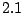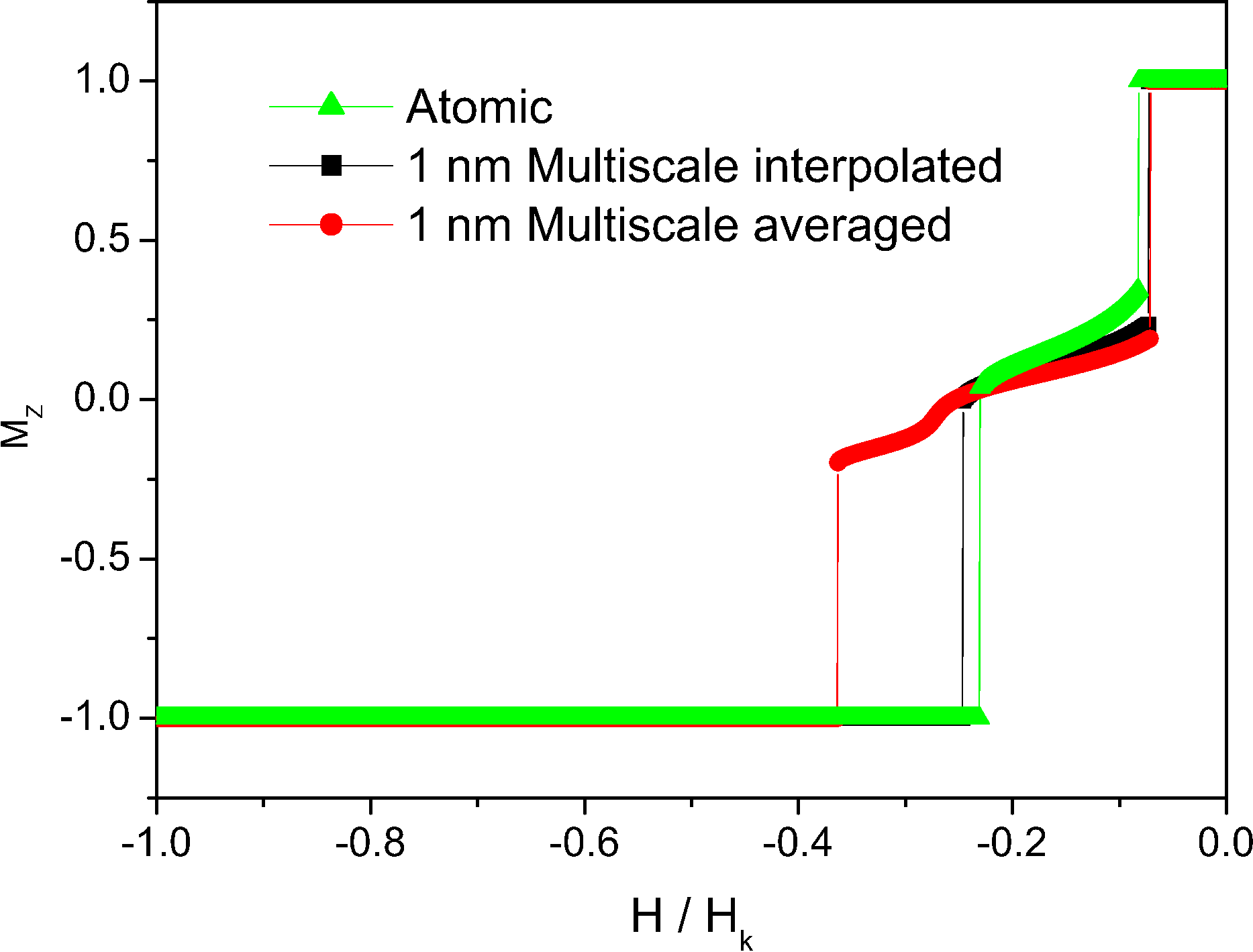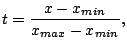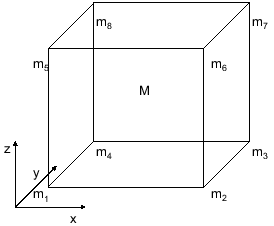## 2.2.4 Passing atomistic information to micromagnetics: a multiscale model2.1

The multiscale calculations involve the use of several models of different length scales in the same calculations. There is more than one approach to include the ab-initio, atomistic and micromagnetic (mesoscopic) calculations. Unfortunately, the simulations can not reach the scale of real samples, that is up to, but it is able to simulate the small size systems produced by nowadays technology. As a first example of multiscale modeling we examine again the work of O.N. Mryasov [Mryasov 05]. The motivation of the work is the temperature dependence of the anisotropy of FePt. In the case of a two ion anisotropy Skomski et al. have shown that the Callen-Callen law has to be changed by adependence [Skomski 03b]. The experimental factor in the low-temperature range in FePt is[Okamoto 02,Thiele 02]. From Langevin Dynamics atomistic calculations using the effective Hamiltonian (see Eq. (2.1)) with the parameters obtained from ab-initio it was possible to reproduce this temperature dependence. Posteriorly, based on the same model D. Hinzke [Hinzke 07] has calculated the temperature dependence of the domain wall width, thus obtaining the micromagnetic exchange parameter. Those data can be included in a micromagnetic simulation to obtain values at finite temperature and this procedure is equivalent to the method shown in Fig. 2.1.Other method, in parallel to the previous one, will be used in the next chapter to calculate magnetization processes in soft/hard bilayers. The necessity of the multiscale approach for small intergranular exchange values has been suggested in Refs. [Kronmüller 97,Kronmüller 01]. The multiscale model is based on the partitioning of the computational cell into atomistic and micromagnetic regions, as shown schematically in Fig. 2.5. The atomistic scale discretization is used in the interface regions, where spatially rapid changes in magnetization might be expected. In this region, the exchange is treated exactly within the Heisenberg model, allowing the system to access to the entire spectrum of magnetic excitations. The lattice structure is taken into account explicitly. For the calculation of the magnetostatic field, the atomistic region is partitioned into macrocells'' of the same size as the micromagnetic region. Inside each macrocell the volume charges are neglected. For computational simplicity the thickness of the atomistic region is commensurate with the size of the micromagnetic cells. The average magnetization of each macrocell is calculated and used in the micromagnetic evaluation of the magnetostatic field. The magnetostatic field is calculated using DADI. Finally, we have to consider the interface between the atomistic and micromagnetic region. On this boundary we first used [Garcia-Sanchez 05] Heisenberg exchange between the actual atoms in the atomistic region and ‘virtual’ atoms in the micromagnetic region having the direction of the average magnetization in the micromagnetic cell projected onto the physical lattice, corresponding to the gray region in Fig. 2.5.The averaging method to obtain the micromagnetic macrocells from atomistic moments has drawbacks, as for example in the case of the hysteresis loops presented in Fig. 2.6 those correspond to FePt/FeRh bilayer with easy axis perpendicular to the plane (Z direction) and applied field in the Z direction and dimensions. The calculation using averaging overestimates the coercive field due to the fact that averaging does not result in a continuous variation of the magnetization. In this case the best method is the interpolation of the micromagnetic moment from the nearest atomistic moments. The used method is a trilinear interpolation. The spatial coordinates are expressed as:(2.35)(2.36)(2.37)

and the magnetization is obtained from the following equation:

 (2.38)

The notations are shown in Fig. 2.7. The interpolation gives the proper micromagnetic magnetization values at the center. After interpolation we obtain the correct coercive field as we can see in Fig. 2.6.2008-04-04